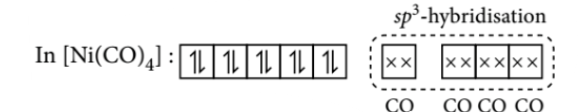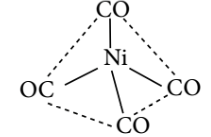Valence bond theory
Question

# The geometry and magnetic behaviour of the complex $\left[\mathrm{Ni}\left(\mathrm{CO}{\right)}_{4}\right]$ are

Moderate
Solution

## $\mathrm{Ni}\left(28\right):\left[\mathrm{Ar}\right]3{d}^{8}4{s}^{2}$Thus, the complex is $s{p}^{3}$ hybridised with tetrahedral geometry and diamagnetic in nature.Get Instant Solutions